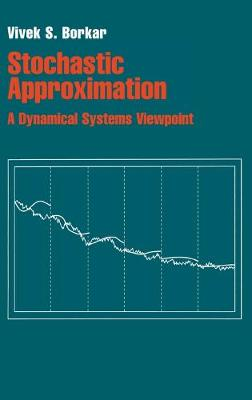•Stochastic Approximation: A Dynamical Systems Viewpoint (Hardback)

(author)
£49.99
Hardback 176 Pages / Published: 01/09/2008
• Not available

This product is currently unavailable.

This simple, compact toolkit for designing and analyzing stochastic approximation algorithms requires only basic literacy in probability and differential equations. Yet these algorithms have powerful applications in control and communications engineering, artificial intelligence and economic modelling. The dynamical systems viewpoint treats an algorithm as a noisy discretization of a limiting differential equation and argues that, under reasonable hypotheses, it tracks the asymptotic behaviour of the differential equation with probability one. The differential equation, which can usually be obtained by inspection, is easier to analyze. Novel topics include finite-time behaviour, multiple timescales and asynchronous implementation. There is a useful taxonomy of applications, with concrete examples from engineering and economics. Notably it covers variants of stochastic gradient-based optimization schemes, fixed-point solvers, which are commonplace in learning algorithms for approximate dynamic programming, and some models of collective behaviour. Three appendices give background on differential equations and probability.

Publisher: Cambridge University Press
ISBN: 9780521515924
Number of pages: 176
Weight: 380 g
Dimensions: 233 x 157 x 17 mm

MEDIA REVIEWS
'I highly recommend [this book] to all readers interested in the theory of recursive algorithms and its applications in practice.' Mathematical Reviews
'This simple compact toolkit for designing and analyzing stochastic approximation algorithms requires only basic literacy in probability and differential equations ... Ideal for graduate students, researchers and practitioners in electrical engineering and computer science, especially those working in control, communications, signal processing and machine learning, this book is also relevant to economics, probability and statistics.' L'Enseignement Mathematique
"I highly recommend it to all readers interested in the theory of recursive algorithms and its applications in practice." Oleg N. Granichin, Mathematical Reviews